Next: Modified Miller dendrites Up: Dendrites Previous: Miller dendrite

## Dendrites of de Groot-Wille type

An example of a rigid dendrite has been roughly described in [J. de Groot et al. 1958]. Its construction is recalled below (see also [Charatonik 1979, Chapter III, Section 5 , p. 227]).

For any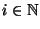let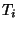denote the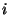-od being the union ofstraight line unit segments emanating from a point called the origin of. We proceed by induction. Define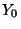as the unit segment. Let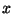be the midpoint of, and define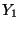as the union ofand a diminished copy of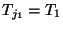so that the diameter of this copy is less than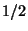and that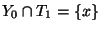. Assume that a tree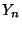is defined such that it is the union of finitely many straight line segments and contains (properly diminished) copies of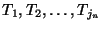, i.e., of the first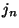terms of the sequence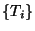. To define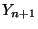consider all maximal free segments in. Note that there are finitely many, say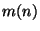of them. Letdenote the midpoint of any of these segments. With each pointso defined we associate, in a one-to-one way, a settaken from theconsecutive terms of the sequence, i.e., we use in this step of the construction the sets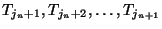, where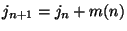. We take each midpoint as the origin of a properly diminished copy of, where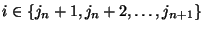in such a way that the diameter of the copy ofis less than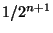and thathas only the pointin common with the attached copy of. All this can clearly be done so carefully that the resulting setis a tree and the limit continuum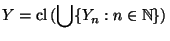is a dendrite. See Figure A.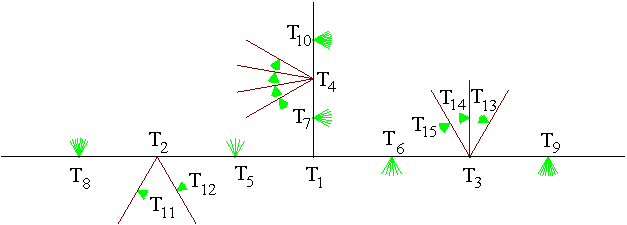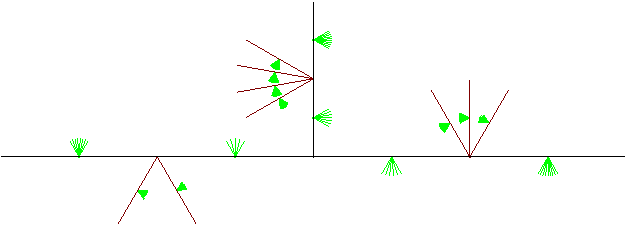The following properties of the dendrite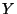are shown in [Charatonik 1979, Statement 10, p. 229].

1. The set of all end points ofis dense in.
2. For each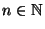the set of all points ofthat are of order at least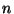is dense in.
3. For each natural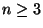the dendritecontains exactly one point of order.
4. For each point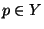and for each component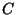of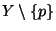the continuum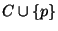contains a homeomorphic copy of.
5.is not strongly rigid.
6.is chaotic.

Recall that for any dendrite conditions 1 and 3 imply 5 and 6, see [Charatonik 1999, Theorem 13, p. 22]. However, neither the above mentioned conditions, nor a rough description given in [J. de Groot et al. 1958], nor the one presented in [Charatonik 1979] lead to a uniquely determined dendrite, because the constructed dendritedepends on a function that assigns the consecutive-ods(used in the succesive steps of the construction) to the midpoints of the maximal free arcs in the trees. Thus we refer to any of the dendritesobtained in this way as to a dendrite of de Groot-Wille type .

An example of a dendrite of de Groot-Wille type that is chaotic but not openly chaotic is constructed in [Charatonik 2000, Theorem 3.10, p. 646].

Here you can find source files of this example.

Here you can check the table of properties of individual continua.

Here you can read Notes or write to Notes ies of individual continua.Next: Modified Miller dendrites Up: Dendrites Previous: Miller dendrite
Janusz J. Charatonik, Pawel Krupski and Pavel Pyrih
2001-11-30